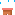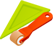Discovery Of Science# Answering skill ( Section B )

Answering skill ( Section B )
 Question How to answer/Example Constant variable 须固定的事项 i. What to keep the same? ii. What is the constant variable?iii. What is the controlled variable?iv. State one controlled variable. (Constant variable) Same Manipulated variable 须改变的事项 i. What to be change?ii. What is the manipulated variable?iii. State one manipulated variable. (Manipulated variable)different Responding variable 须观察的事项 i. What to be observe?ii. What is the responding variable?iii. State one responding     variable (Responding variable)Observed and recorded Aim / Purpose目的 i. What is the aim of the   experiment/ investigating?ii. What is the purpose of the experiment/ investigating?iii. State the aim/purpose the experiment/ investigating. To investigate the relationship between (Manipulated variable) with/and/towards/upon/on (Responding variable).  Ex: To investigate the  relationship between the shape of paper (mv)and the ability to support books(rv). Inferences推断 i. Give the reason.ii. State why. Because_______________ Ex: because more hunting activity /destruction of forest. Hypothesis假设 i.  What is the hypothesis of the investigation/experiment? i. When(Manipulated variable)increases/decreases the (Responding variable)increases/decreases.ii. If (Manipulated variable)…, so(Responding variable)… .iii. More (Manipulated variable), more(Responding variable).Ex: i. More sugar will dissolve in greater amount of water.ii. Sugar will dissolve more quickly in a greater amount of water. Conclusion结论 i. What is the conclusion can be made?ii. State one conclusion. i. More (Manipulated variable), more(Responding variable).Ex: The more battery used, the faster the car goes. Relationship关系 i. State the relationship between  manipulated variable and responding variable. i.  More (Manipulated variable), more(Responding variable).ii. When(Manipulated variable)increases/decreases the (Responding variable)increases/decreases.Ex: i.The more battery used, the faster the car goes.ii. When more battery are used, the speed of car increases.iii. As the temperature increases more sugar will dissolve. Observe观察 i. State one observation based on the investigation. (your observation, based the picture or table)i.  ______most, next_______,________.Ex: The water temperature in beaker Z dropped the most, next is beaker X. Beaker Y’s temperature remains constant. Pattern /trend变化形式 i.  What is the trend of change in the number of…ii. What is the pattern of change in the number of… Increases 上升 / decreases下降/ unchanged 保持不变